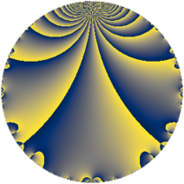# Properties

 Label 19.2.aLevel $19$ Weight $2$ Character orbit 19.a Rep. character $\chi_{19}(1,\cdot)$ Character field $\Q$ Dimension $1$ Newform subspaces $1$ Sturm bound $3$ Trace bound $0$

# Related objects

## Defining parameters

 Level: $$N$$ $$=$$ $$19$$ Weight: $$k$$ $$=$$ $$2$$ Character orbit: $$[\chi]$$ $$=$$ 19.a (trivial) Character field: $$\Q$$ Newform subspaces: $$1$$ Sturm bound: $$3$$ Trace bound: $$0$$

## Dimensions

The following table gives the dimensions of various subspaces of $$M_{2}(\Gamma_0(19))$$.

Total New Old
Modular forms 2 2 0
Cusp forms 1 1 0
Eisenstein series 1 1 0

The following table gives the dimensions of the cuspidal new subspaces with specified eigenvalues for the Atkin-Lehner operators and the Fricke involution.

$$19$$Dim
$$-$$$$1$$

## Trace form

 $$q - 2 q^{3} - 2 q^{4} + 3 q^{5} - q^{7} + q^{9} + O(q^{10})$$ $$q - 2 q^{3} - 2 q^{4} + 3 q^{5} - q^{7} + q^{9} + 3 q^{11} + 4 q^{12} - 4 q^{13} - 6 q^{15} + 4 q^{16} - 3 q^{17} + q^{19} - 6 q^{20} + 2 q^{21} + 4 q^{25} + 4 q^{27} + 2 q^{28} + 6 q^{29} - 4 q^{31} - 6 q^{33} - 3 q^{35} - 2 q^{36} + 2 q^{37} + 8 q^{39} - 6 q^{41} - q^{43} - 6 q^{44} + 3 q^{45} - 3 q^{47} - 8 q^{48} - 6 q^{49} + 6 q^{51} + 8 q^{52} + 12 q^{53} + 9 q^{55} - 2 q^{57} - 6 q^{59} + 12 q^{60} - q^{61} - q^{63} - 8 q^{64} - 12 q^{65} - 4 q^{67} + 6 q^{68} + 6 q^{71} - 7 q^{73} - 8 q^{75} - 2 q^{76} - 3 q^{77} + 8 q^{79} + 12 q^{80} - 11 q^{81} + 12 q^{83} - 4 q^{84} - 9 q^{85} - 12 q^{87} + 12 q^{89} + 4 q^{91} + 8 q^{93} + 3 q^{95} + 8 q^{97} + 3 q^{99} + O(q^{100})$$

## Decomposition of $$S_{2}^{\mathrm{new}}(\Gamma_0(19))$$ into newform subspaces

Label Dim $A$ Field CM Traces A-L signs $q$-expansion
$a_{2}$ $a_{3}$ $a_{5}$ $a_{7}$ 19
19.2.a.a $1$ $0.152$ $$\Q$$ None $$0$$ $$-2$$ $$3$$ $$-1$$ $-$ $$q-2q^{3}-2q^{4}+3q^{5}-q^{7}+q^{9}+3q^{11}+\cdots$$